# Quiz 11: Investment Analysis and Taxation of Income Properties

Business

a. The EGI (Effective Gross Income) from a rental property is the actual amount of rent expected to be collected after allowing for the vacancy. The computation of EGI provided below. Answers are highlighted in 'Orange'. Use spreadsheet for the ease in computation. Enter given values and formulas in the spreadsheet as shown in the image below. In Cells A1:H38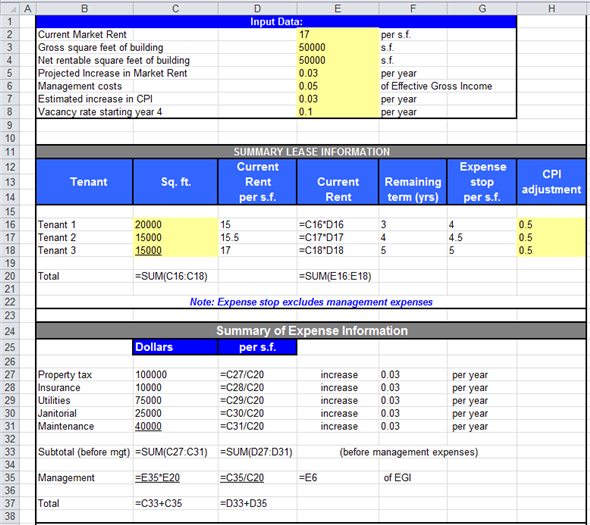In Cells A39:D65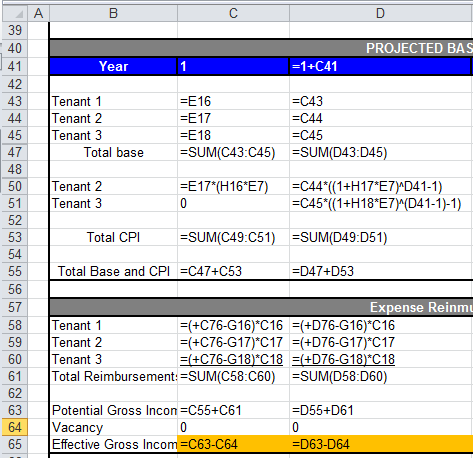In cells E39:H65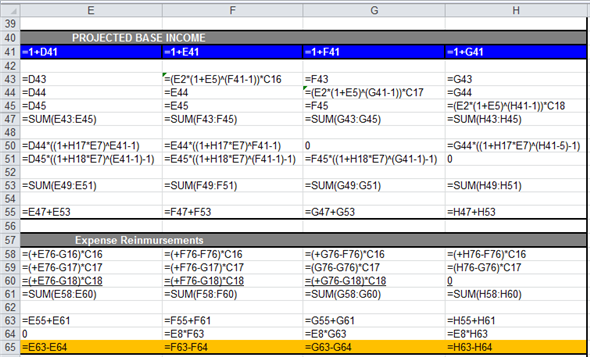Obtained result is provided below.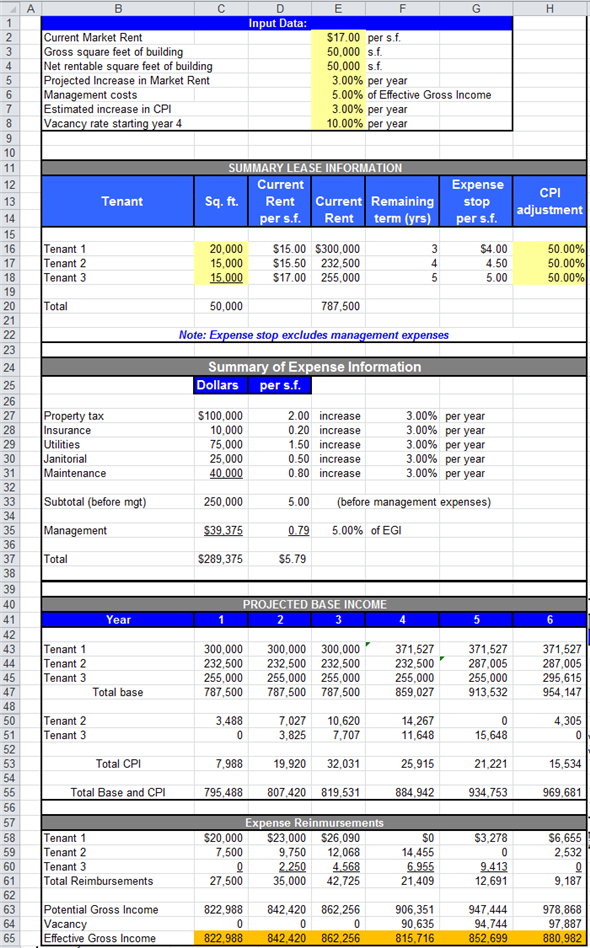b. Generally, operating expenses are paid by the owner of the building not by the tenant, but if there is condition like stop expense then over and above of stop expenses will be paid by tenant. This payment of operating expense by tenant is called expense reimbursement. Enter given values and formulas in the spreadsheet as shown in the image below.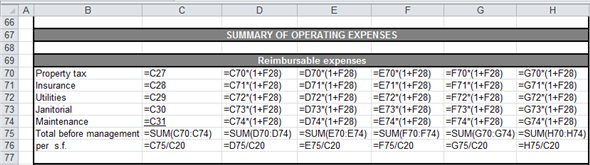Obtained results are provided below.c. Net operating income (NOI) is the income or profit which is generated from investing in a project or any other investment avenues. It is calculated as the revenue earned from the property after adjusting the operating expenses associated with the property. A property's projected net operating income ( NOI ) is dependent on several factors like the expected occupancy rate in future, the expected rent in the market in future etc. The computation of NOI is provided below. Enter given values and formulas in the spreadsheet as shown in the image below.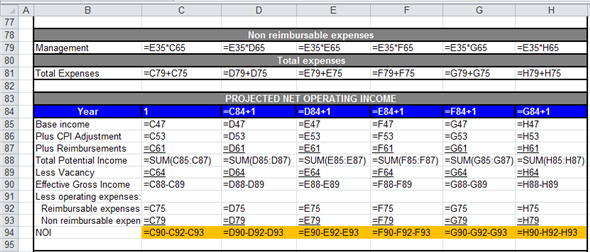Obtained results are provided below.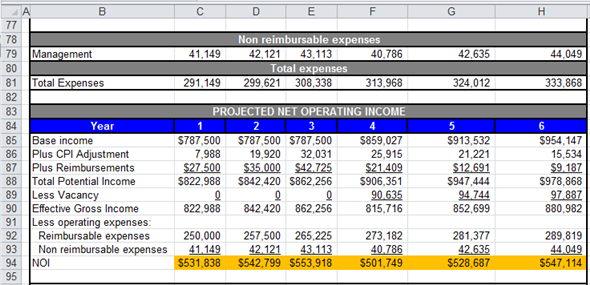d. The average compound rate can be defined as the rate at which the NOI (Net Operating Income) increase from beginning year to terminal year. Computation of average compound rate is provided below. Enter given values and formulas in the spreadsheet as shown in the image below.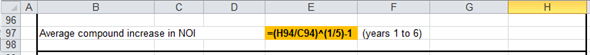Obtained result is provided below.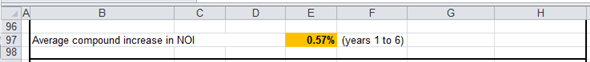Thus, the average compound rate is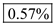. e. The going-in capitalization rate is the ratio of purchase price and NOI (Net Operating Income) of first-year. The computation of capitalization rate is provided below. Enter given values and formulas in the spreadsheet as shown in the image below.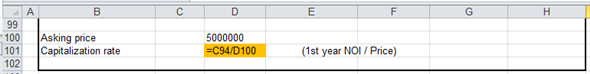Obtained result is provided below.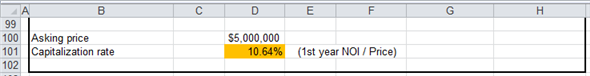Thus, the capitalization rate is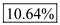.

Investment: Investment refers to the utilization of funds in financial instruments or physical items which can be acquired and traded without any difficulty in the free market. It includes securities, commodities and real estate property. Investment in real estate means venture in land or a building property. The primary benefits from investing in real estate income property: • Investment in real estate will provide evade against inflation which will result in increase in the value of property and rental value. • It also provides tax benefit as rental income is tax exempt. • Real estate investment is a long term investment and value of asset will increase with time. • Investor have the decision making power, as investor will decide the amount of rent to be charged from the tenant. It also provides steady income. Hence, the primary benefits from investing in real estate income property are real estate appreciation, steady income and financial security.

Net Present Value is a capital budgeting technique, which takes into account the time value of money. Its aim is the maximization of the shareholder's profit. NPV is defined as the difference between the PV of cash inflows and cash outflows. NPV is the opportunity rate of return to the investors. If the NPV of the project is positive it may be accepted, if negative it will be rejected and in case, zero, corporation may be indifferent. The formula for Net Present Value is: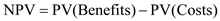a. An employee of University computes NPV. Given, Price of property \$1,250,000, rent estimate is \$200,000 growing at 3%. Vacancies loss is 10%, operating expenses are 35% and the discount rate is 12% and 9%. Computation of IRR is done through Excel sheet by using interpolation method as presented below. The computation of cash flows is as below.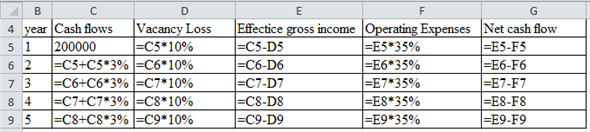The result of the above excel sheet is as below.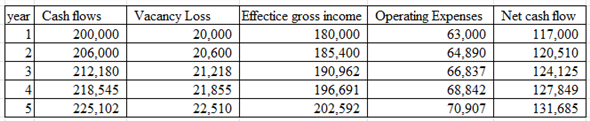The computation of BTIRR is as below.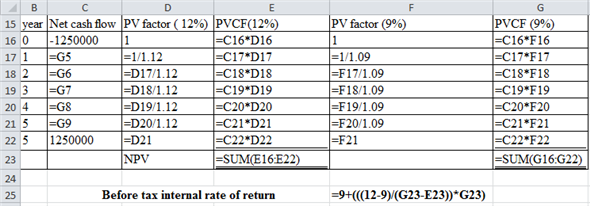The result of the above excel sheet is as below.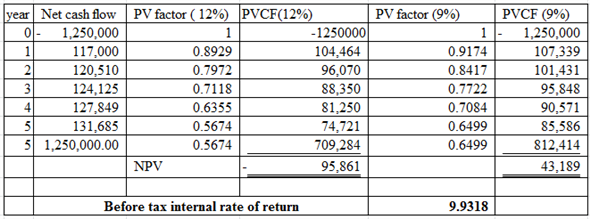The internal rate of return before tax is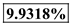. b. Debt coverage ratio: It measures the availability of cash flow to full fill the current debt obligation. It is computed by simply dividing the operating income by the current debt. Computation of debt coverage ratios for the first year is as below.The debt coverage ratio is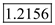when we consider that the total loan amount will be refunded at the end of the term. c. For the estimation of scrap value or residual value at the end of holding period, the terminal capitalization rate is used. It can be computed by dividing the net operating income by the terminal value of the asset. In the given situation the terminal residual value of the asset is computed as below.Terminal value of the property is equal to \$14,49,093. Here, the Net operating incomes are different for different years, so terminal capitalization rate also differ for different years. d. NPV is computed by using a discount rate of 14% is computed as below. The computation is done through the excel as presented below.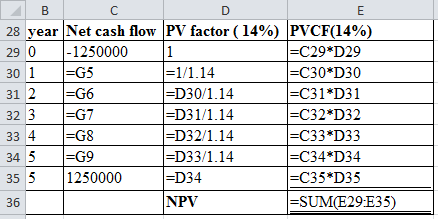The result of the above excel sheet is as below.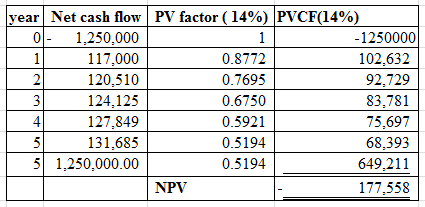Net present value is negative when the discounted rate is 14%, and it signifies that the present value of cash inflows is less than the initial cash outflow. When the discount rate of 14% is considered then the project is not accepted. e. Profitability index is computed by dividing the future value of cash flows by the initial investment. Using 14% discount rate the profitability index is computed as below.Profitability index of the project, using 14% discount factor is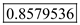. This rate is below one, hence not profitable in nature. This rate is computed for the purpose of ranking of different projects.